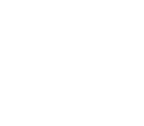# arcLeft

Category:Turtle

Category: Turtle

Moves the turtle forward and to the left in a smooth, circular arc.

The turtle is not limited to only moving in a straight line. arcLeft(angle,radius) moves the turtle counterclockwise along an angle degree arc of a radius sized circle. The center of the circle is radius pixels to the left of the starting turtle position and direction.

### Examples

```// Draw a quarter circle counterclockwise.
arcLeft(90, 25);
```

Example: One Ring to Rule Them All Draw a full circle counterclockwise.

```// Draw a full circle counterclockwise.
penColor("gold");
penWidth(15);
arcLeft(360, 50);
```

Example: Negative Angle arcLeft always moves the turtle counterclockwise. For a negative angle the turtle moves (360+angle) degrees.

```// arcLeft always moves the turtle counterclockwise.
// For a negative angle the turtle moves (360+angle) degrees.
arcLeft(-45, 100);
```

Example: Spiral Spiral into the center of a circle.

 ```// Spiral into the center of a circle. for (var radius=50; radius>0; radius=radius-5) { arcLeft(180, radius); } ```### Syntax

```arcLeft(angle, radius);
```

### Parameters

Name Type Required? Description
angle number Yes The angle degree arc to move the turtle counterclockwise in a circle.
radius number Yes The radius of the circle that is placed left of the turtle. radius must be >= 0.

### Returns

No return value. Moves turtle only.

### Tips

• Use penUp() before calling arcLeft() to have the turtle not draw as it moves.
• You can specify a radius of 0, which makes arcLeft() act the same as turnLeft().
• Use alternating with arcRight() to make wavy lines.

Found a bug in the documentation? Let us know at documentation@code.org

Found a bug in the documentation? Let us know at documentation@code.org